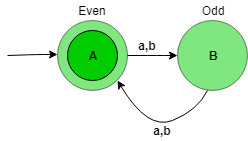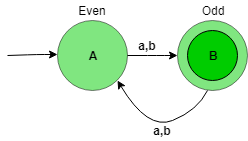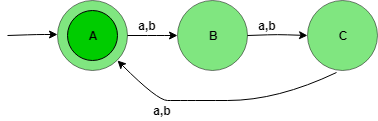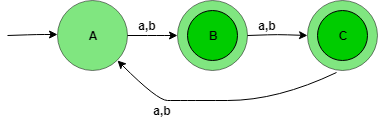GeeksforGeeks App
Open AppBrowser
Continue

# Designing Deterministic Finite Automata (Set 2)

Prerequisite – Designing finite automata, previous article: Designing Deterministic Finite Automata (Set 1)
In this article, we will see some designing of Deterministic Finite Automata (DFA).

Problem-1: Construction of a DFA for the set of string over {a, b} such that length of the string |w| is divisible by 2 i.e, |w| mod 2 = 0.

Explanation – The desired language will be like:

`L = {?, aa, ab, ba, bb, aaaa, bbbb, ............} `

The state transition diagram of the language will be like:Here, state A represent set of all string of length even (0, 2, 4, …), and state B represent set of all string of length odd (1, 3, 5, …).

```Number of states: n
If |W| mod n = 0 ```

 `def` `stateA(n):``    ``if``(``len``(n)``=``=``0``):``        ``print``(``"Accepted"``)``    ``else``:  ``         ` `        ``#on any input call function stateB``        ``if` `(n[``0``]``=``=``'0'` `or` `n[``0``]``=``=``'1'``):``            ``stateB(n[``1``:])``         ` `def` `stateB(n):``    ``if``(``len``(n)``=``=``0``):``        ``print``(``"Not Accepted"``)``    ``else``:``         ` `         ``#on any input call function stateA``        ``if` `(n[``0``]``=``=``'0'` `or` `n[``0``]``=``=``'1'``):``            ``stateA(n[``1``:])        `` ` ` ` ` ` `#take input``n``=``input``()`` ` `#call stateA``#to check the input``stateA(n)`

The above automata will accept all the strings having the length of the string divisible by 2. When the length of the string is 1, then it will go from state A to B. When the length of the string is 2, then it will go from state B to A and so on. State A is the final state i.e, it accept all the string having length divisible by 2.

Problem-2: Construction of a DFA for the set of string over {a, b} such that length of the string |w| is not divisible by 2 i.e, |w| mod 2 = 1.

Explanation – The desired language will be like:

`L = {a, b, aaa, aab, aba, abb, aaaaa, bbbb, .......} `

The state transition diagram of the language will be like:Here, state A represent set of all string of length even (0, 2, 4, …), and state B represent set of all string of length odd (1, 3, 5, …).

 `def` `stateA(n):``    ``if``(``len``(n)``=``=``0``):``        ``print``(``"Not Accepted"``)``    ``else``:  ``         ` `        ``#on any input call function stateB``        ``if` `(n[``0``]``=``=``'0'` `or` `n[``0``]``=``=``'1'``):``            ``stateB(n[``1``:])``         ` `def` `stateB(n):``    ``if``(``len``(n)``=``=``0``):``        ``print``(``"Accepted"``)``    ``else``:``         ` `         ``#on any input call function stateA``        ``if` `(n[``0``]``=``=``'0'` `or` `n[``0``]``=``=``'1'``):``            ``stateA(n[``1``:])        `` ` ` ` ` ` `#take input``n``=``input``()`` ` `#call stateA``#to check the input``stateA(n)`

The above automata will accept all the strings having the length of the string not divisible by 2. When the length of the string is 1, then it will go from state A to B. When the length of the string is 2, then it will go from state B to A and so on. State B is the final state i.e, it accept all the string having length not divisible by 2.

Problem-3: Construction of a DFA for the set of string over {a, b} such that length of the string |w| is divisible by 3 i.e, |w| mod 3 = 0.

Explanation – The desired language will be like:

`L = {?, aaa, aab, aba, abb, aaaaaa, bbbbbb, .......} `

The state transition diagram of the language will be like:Here, state A represents set for which string’s length divided by 3 then remainder is zero (0), state B represents set for which string’s length divided by 3 then the remainder is one (1), and state C represents set for which string’s length divided by 3 then the remainder is two (2).

```Number of states: n
If |W| mod n = 0 ```

 `def` `stateA(n):``    ``if``(``len``(n)``=``=``0``):``        ``print``(``"Accepted"``)``    ``else``:  ``         ` `        ``#on any input call function stateB``        ``if` `(n[``0``]``=``=``'0'` `or` `n[``0``]``=``=``'1'``):``            ``stateB(n[``1``:])``         ` `def` `stateB(n):``    ``if``(``len``(n)``=``=``0``):``        ``print``(``"Not Accepted"``)``    ``else``:``         ` `         ``#on any input call function stateC``        ``if` `(n[``0``]``=``=``'0'` `or` `n[``0``]``=``=``'1'``):``            ``stateC(n[``1``:])  ``             ` `def` `stateC(n):``    ``if``(``len``(n)``=``=``0``):``        ``print``(``"Not Accepted"``)``    ``else``:``         ` `         ``#on any input call function stateA``        ``if` `(n[``0``]``=``=``'0'` `or` `n[``0``]``=``=``'1'``):``            ``stateA(n[``1``:])              `` ` ` ` ` ` `#take input``n``=``input``()`` ` `#call stateA``#to check the input``stateA(n)`

The above automata will accept all the strings having the length of the string divisible by 3. When the length of the string is 1, then it will go from state A to B. When the length of the string is 2, then it will go from state B to C and When the length of the string is 3, then it will go from state C to A (final state). State A is the final state i.e, it accepts all the string having the length divisible by 3.

Problem-4: Construction of a DFA for the set of string over {a, b} such that length of the string |w| is not divisible by 3 i.e, |w| mod 3 = 1.

Explanation – The desired language will be like:

`L = {a, b, aa, ab, ba, bb, aaaa, bbbb, ........} `

The state transition diagram of the language will be like:Here, state A represents set for which string’s length divided by 3 then remainder is zero (0), state B represents set for which string’s length divided by 3 then the remainder is one (1), and state C represents set for which string’s length divided by 3 then the remainder is two (2).

 `def` `stateA(n):``    ``if``(``len``(n)``=``=``0``):``        ``print``(``"Not Accepted"``)``    ``else``:  ``         ` `        ``#on any input call function stateB``        ``if` `(n[``0``]``=``=``'0'` `or` `n[``0``]``=``=``'1'``):``            ``stateB(n[``1``:])``         ` `def` `stateB(n):``    ``if``(``len``(n)``=``=``0``):``        ``print``(``"Accepted"``)``    ``else``:``         ` `         ``#on any input call function stateC``        ``if` `(n[``0``]``=``=``'0'` `or` `n[``0``]``=``=``'1'``):``            ``stateC(n[``1``:])  ``             ` `def` `stateC(n):``    ``if``(``len``(n)``=``=``0``):``        ``print``(``"Not Accepted"``)``    ``else``:``         ` `         ``#on any input call function stateA``        ``if` `(n[``0``]``=``=``'0'` `or` `n[``0``]``=``=``'1'``):``            ``stateA(n[``1``:])              `` ` ` ` ` ` `#take input``n``=``input``()`` ` `#call stateA``#to check the input``stateA(n)`

The above automata will accept all the strings having the length of the string not divisible by 3. When the length of the string is 1, then it will go from state A to B. When the length of the string is 2, then it will go from state B to C and When the length of the string is 3, then it will go from state C to A. State B and C are the final state i.e, it accepts all the string having the length not divisible by 3.

My Personal Notes arrow_drop_up